# Using VLOOKUP and HLOOKUP Functions

##### In this article, we will demonstrate how to use the VLOOKUP and HLOOKUP Functions in Microsoft Excel.

For dedicated, instructor driven Excel training in Los Angeles call us on 888.815.0604..

Both the VLOOKUP and HLOOKUP functions are used to find specific item(s) in a table of data. The VLOOKUP function contains a number of arguments which are written out like this:

VLOOKUP (value that is being looked for, lookup table name or range, number of the column in the table containing the relevant data, true or false)

To use the VLOOKUP function correctly, you need to have your spreadsheet data laid out properly in table form with at least two columns. The first column in the table will contain the keys (identifiers that the VLOOKUP function will examine for a match). In the sample workbook, the keys are the names of the cities. This first column can be referred to as the lookup column: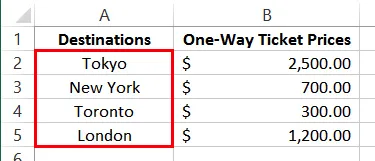The other columns in the table contain data that corresponds to the column of keys. Your table can be several columns wide, and you can specify which column VLOOKUP will retrieve data from by putting a number corresponding to the given column in the function.

You can see that this table contains data about the airfare costs to a number of different cities. To help simplify things, all of the data inside the table has been given the range name “prices:”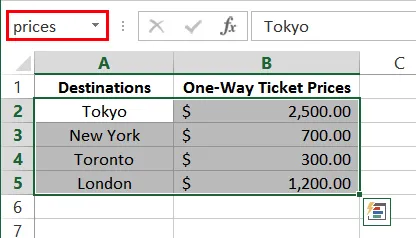Click inside D2 and type =VLOOKUP(“New York”,prices,2) into the formula bar. Press Enter on your keyboard to apply the new formula: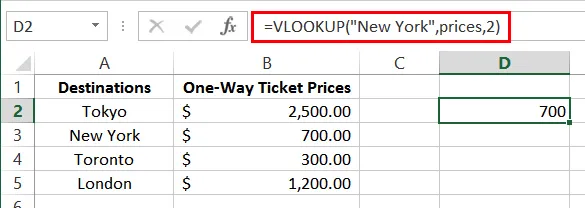D2 will now show the value 700 (the value of a ticket to New York). This lookup function looked vertically down the leftmost column of the lookup table (prices) until it found a match for the text string “New York.” The function then returned the value that is in the second (2) column of the table, in the row where the match was found.

HLOOKUP operates the same as VLOOKUP, except that it looks across rows (horizontal) for a match rather than down (vertical) columns. If you were to use HLOOKUP, you will need to have a table arranged in a horizontal manner, like so: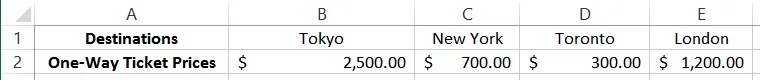If you prefer, you can use the Function Arguments dialog to create VLOOKUP or HLOOKUP functions. Click Formulas → Lookup & Reference → VLOOKUP (or HLOOKUP):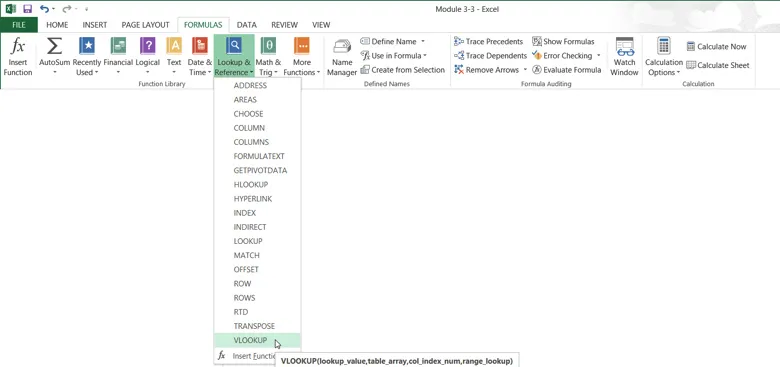Then, you can use the Function Arguments dialog to construct your function.

#### Class based Microsoft Excel available in Los Angeles

Join our Microsoft Excel training workshop. We run MS classes for all skill levels, every month. Check out our extensive student testimonials Excel class reviews.